### Featured

October 22, 2020

In my last post I touched on the topic of continuously improving your geo-computing projects (also take a look at my chapter from the upcoming Software Underground book, 52 things you should know about geocomputing).

However, one aspect that I intentionally left out in was that of coding skills as I was planning to get back to it with a dedicated post, which you are reading just now.

#### 2018 vs 2020 comparison of flag percentage calculation

In the Jupyter notebook I compare the results of seismic inversion from two methods (with or without inversion-tailored noise attenuation) using a custom function to flag poor prediction of the target well log using median/median absolute deviation as a statistic for the error; the results are shown below.

One may just do this visual comparison, but I also included calculations to count the number and percentage of samples that have been flagged for each case. Below is a cell of code from the Jupyter notebook (let’s call it 2020 code) that does just that .

``zone_errors_a['flagged samples']=result_a.groupby('zone', sort=False).flag.sum().valueszone_errors_b['flagged samples']=result_b.groupby('zone', sort=False).flag.sum().valuesdef calc_proportion(dtf):    """    function to calculate proportion of flagged samples    """    x=dtf.flag    return round(100 * x.sum()/len(x), 1)zone_errors_a['proportion (%)']=result_a.groupby('zone',sort=False).apply(calc_proportion).valueszone_errors_b['proportion (%)']=result_b.groupby('zone',sort=False).apply(calc_proportion).values``

I am a lot happier with this code than with the original code (circa 2018), which is in the cell below.

``````zones_a=list(result_a['zone'].unique())
zones_b=list(result_b['zone'].unique())

zone_errors_a['flagged samples']=[result_a.loc[result_a.zone==z,'flag'].sum() for z in zones_a]
zone_errors_b['flagged samples']=[result_b.loc[result_b.zone==z,'flag'].sum() for z in zones_b]

zone_errors_a['proportion (%)']=[round(result_a.loc[result_a.zone==z,  'flag'].sum()/len(result_a.loc[result_a.zone==z,'flag'])*100,1) for z in zones_a]

zone_errors_b['proportion (%)']=[round(result_b.loc[result_b.zone==z,  'flag'].sum()/len(result_b.loc[result_b.zone==z,'flag'])*100,1) for z in zones_b]                                    ``````

The major differences in the older code are:

• I was using `unique` instead of Pandas’ `groupby`
• I was using list comprehensions to work through the DataFrame, instead of Pandas’ `apply` and a custom function to calculate the percentages on the entire DataFrame at once.

I find the 2020 code much more tidy and easier to read.

#### Enters Pandas for everyone

The above changes happened in but a few hours over two evenings, after having worked through chapters 9 and 10 of Pandas for Everyone by Daniel Chen, a very accessible read for all aspiring data scientists, which I highly recommend (also, watch Daniel’s fully-packed 2019 Pycon tutorial).

And before you ask: no, you do not get the Agile Scientific sticker with the book, I am sorry.

🙂

#### Comparison of 2016 vs 2020 code snippets from the 2016 SEG Machine Learning contest

A second example is of code used to calculate the first and second derivatives for all geophysical logs from the wells in the 2016 SEG Machine Learning contest.

The two cells of code below do exactly the same thing: loop through the wells and for each one in turn loop through the logs, calculate the derivatives, add them to a temporary Pandas DataFrame, then concatenate into a single output DataFrame. In this case, the only difference is the moving away from `unique` to `groupby`.

I use the `%%timeit` cell magic to compare the runtimes for the two cells.

###### 2016 code
``````%%timeit
# for training data
# calculate all 1st and 2nd derivative for all logs, for all wells
train_deriv_df = pd.DataFrame()             # final dataframe

for well in train_data['Well Name'].unique():        # for each well
new_df = pd.DataFrame() # make a new temporary dataframe

for log in ['GR', 'ILD_log10', 'DeltaPHI', 'PHIND' ,'PE']: # for each log
# calculate and write to temporary dataframe
new_df[str(log) + '_d1'] = np.array(np.gradient(train_feat_df[log][train_feat_df['Well Name'] == well]))

# append all rows of temporary dataframe to final dataframe
train_deriv_df = pd.concat([train_deriv_df, new_df])

86 ms ± 1.47 ms per loop (mean ± std. dev. of 7 runs, 10 loops each)``````
###### 2020 code
``````%%timeit
# for training data
# calculate all 1st and 2nd derivative for all logs, for all wells
train_deriv_df = pd.DataFrame() # final dataframe

for _, data in train_feat_df.groupby('Well Name'): # for each well
new_df = pd.DataFrame()                        # make a new temporary dataframe

for log in ['GR', 'ILD_log10', 'DeltaPHI', 'PHIND' ,'PE']: # for each log
# calculate and write to temporary dataframe

# append all rows of temporary dataframe to final dataframe
train_deriv_df = pd.concat([train_deriv_df, new_df])

52.3 ms ± 353 µs per loop (mean ± std. dev. of 7 runs, 10 loops each)``````

We go down to 52.3 ms from 86 ms, which is a modest improvement, but certainly the code is more compact and a whole lot lighter to read (i.e. more pythonic, or pandaish if you prefer): I am happy!

As an aside, if you want to know more about timing code execution, see section 1.07 from Jake VanderPlas’ outstanding Python Data Science Handbook, which I also cannot recommend enough (and do yourself a favor: watch his series Reproducible Data Analysis in Jupyter).

By the way, below I show the notebook code comparison generated using the nbdiff-web option from the awesome `nbdime` library, a recent discovery.

# Geoscience Machine Learning bits and bobs – data completeness

#### 2016 Machine learning contest – Society of Exploration Geophysicists

In a previous post I showed how to use `pandas.isnull` to find out, for each well individually, if a column has any null values, and `sum` to get how many, for each column. Here is one of the examples (with more modern, pandaish syntax compared to the example in the previous post:

```for well, data in training_data.groupby('Well Name'):
print(well)
print (data.isnull().values.any())
print (data.isnull().sum(), '\n')
```

Simple and quick, the output showed met that  – for example – the well ALEXANDER D is missing 466 samples from the PE log:

```ALEXANDER D
True
Facies         0
Formation      0
Well Name      0
Depth          0
GR             0
ILD_log10      0
DeltaPHI       0
PHIND          0
PE           466
NM_M           0
RELPOS         0
dtype: int64```

A more appealing and versatile alternative, which I discovered after the contest, comes with the `matrix` function form the missingno library. With the code below I can turn each well into a Pandas DataFrame on the fly, then a missingno matrix plot.

```for well, data in training_data.groupby('Well Name'):

msno.matrix(data, color=(0., 0., 0.45))
fig = plt.gcf()
fig.set_size_inches(20, np.round(len(data)/100)) # heigth of the plot for each well reflects well length
axes=fig.get_axes()
axes.set_title(well, color=(0., 0.8, 0.), fontsize=14, ha='center')```

I find that looking at these two plots provides a very compelling and informative way to inspect data completeness, and I am wondering if they couldn’t be used to guide the strategy to deal with missing data, together with domain knowledge from petrophysics.

Interpreting the dendrogram in a top-down fashion, as suggested in the library documentation, my first thoughts are that this may suggest trying to predict missing values in a sequential fashion rather than for all logs at once. For example, looking at the largest cluster on the left, and starting from top right, I am thinking of testing use of GR to first predict missing values in RDEP, then both to predict missing values in RMED, then DTC. Then add CALI and use all logs completed so far to predict RHOB, and so on.

Naturally, this strategy will need to be tested against alternative strategies using lithology prediction accuracy. I would do that in the context of learning curves: I am imagining comparing the training and crossvalidation error first using only non NaN rows, then replace all NANs with mean, then compare separately this sequential log completing strategy with an all-in one strategy.

# Geoscience Machine Learning bits and bobs – data inspection

If you have not read Geoscience Machine Learning bits and bobs – introduction, please do so first as I go through the objective and outline of this series, as well as a review of the dataset I will be using, which is from the  2016 SEG Machine LEarning contest.

#### *** September 2020 UPDATE ***

Although I have more limited time now, compared to 2016,  I am very excited to be participating in the 2020 FORCE Machine Predicted Lithology challenge. Most new work and blog posts will be about this new contest instead of the 2016 one.

#### ***************************

OK, let’s begin!

With each post, I will add a new notebook to the GitHub repo here. The notebook that goes with this post is  called 01 – Data inspection.

#### Data inspection

The first step after loading the dataset is to create a Pandas DataFrame. With the `describe` method I get a lot of information for free:Indeed, from the the first row in the summary I learn that about 20% of samples in the photoelectric effect column PE are missing.

I can use `pandas.isnull` to tell me, for each well, if a column has any null values, and `sum` to get the number of null values missing, again for each column.

```for well in training_data['Well Name'].unique():
print(well)
w = training_data.loc[training_data['Well Name'] == well]
print (w.isnull().values.any())
print (w.isnull().sum(), '\n')
```

Simple and quick, the output tells met, for example, that the well ALEXANDER D is missing 466 PE samples, and Recruit F9 is missing 12.

However,  the printout is neither easy, nor pleasant to read, as it is a long list like this:

```SHRIMPLIN
False
Facies       0
Formation    0
Well Name    0
Depth        0
GR           0
ILD_log10    0
DeltaPHI     0
PHIND        0
PE           0
NM_M         0
RELPOS       0
dtype: int64

ALEXANDER D
True
Facies         0
Formation      0
Well Name      0
Depth          0
GR             0
ILD_log10      0
DeltaPHI       0
PHIND          0
PE           466
NM_M           0
RELPOS         0
dtype: int64

Recruit F9
True
Facies        0
Formation     0
Well Name     0
Depth         0
GR            0
ILD_log10     0
DeltaPHI      0
PHIND         0
PE           12
NM_M          0
RELPOS        0
dtype: int64
...
...
```

From those I can see that, apart from the issues with the PE log, GR has some high values in SHRIMPLIN, and so on…

All of the above is critical to determine the data imputation strategy, which is the topic of one of the next posts; but first in the next post I will use a number of visualizations of  the data, to examine its distribution by well and by facies, and to explore relationships among variables.

# Geoscience Machine Learning bits and bobs – introduction

#### Bits and what?

After wetting (hopefully) your appetite with the Machine Learning quiz / teaser I am now moving on to a series of posts that I decided to title “Geoscience Machine Learning bits and bobs”.

OK, BUT fist of all, what does ‘bits and bobs‘ mean? It is a (mostly) British English expression that means “a lot of small things”.

Is it a commonly used expression? If you are curious enough you can read this post about it on the Not one-off British-isms blog. Or you can just look at the two Google Ngram plots below: the first is my updated version of the one in the post, comparing the usage of the expression in British vs. US English; the second is a comparison of its British English to that of the more familiar “bits and pieces” (not exactly the same according to the author of the blog, but the Cambridge Dictionary seems to contradict the claim).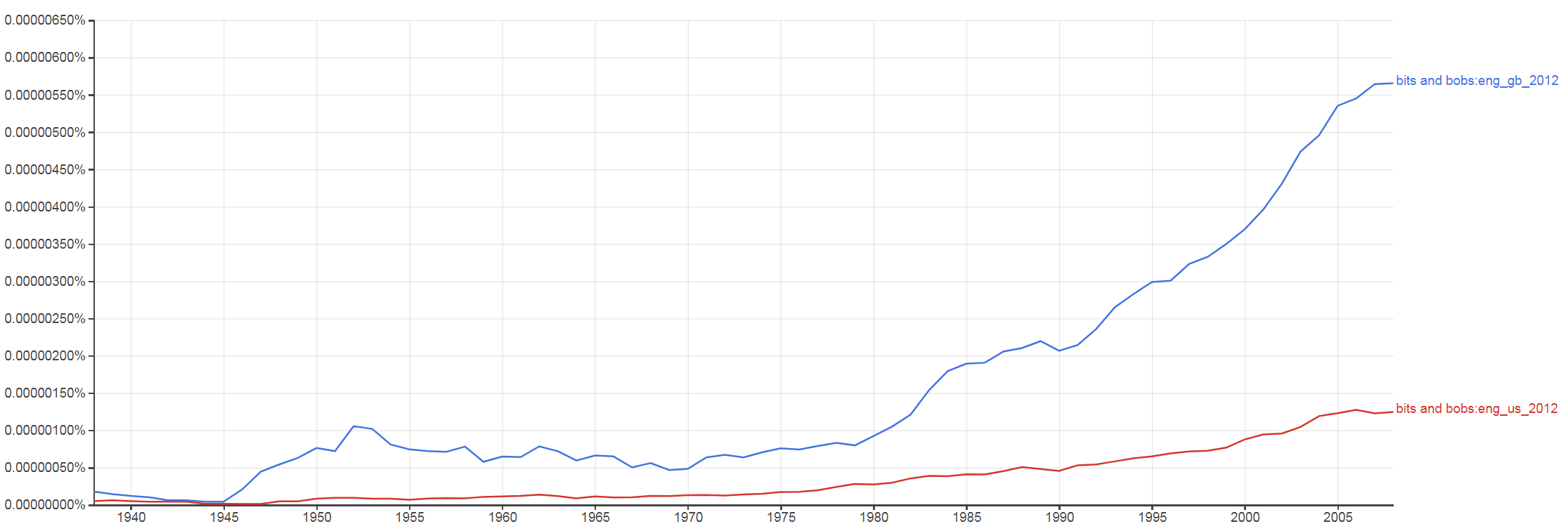I’ve chosen this title because I wanted to distill, in one spot, some of the best collective bits of Machine Learning that came out during, and in the wake of the 2016 SEG Machine Learning contest, including:

• The best methods and insights from the submissions, particularly the top 4 teams
• Things that I learned myself, during and after the contest
• Things that I learned from blog posts and papers published after the contest

I will touch on a lot of topics but I hope that – in spite of the title’s pointing to a random assortment of things –  what I will have created in the end is a cohesive blog narrative and a complete, mature Machine Learning pipeline in a Python notebook.

#### *** September 2020 UPDATE ***

Although I have more limited time these days, compared to 2016,  I am very excited to be participating in the 2020 FORCE Machine Predicted Lithology challenge. Most new work and blog posts will be about this new contest instead of the 2016 one.

#### Some background on the 2016 ML contest

The goal of the SEG contest was for teams to train a machine learning algorithm to predict rock facies from well log data. Below is the (slightly modified) description of the data form the original notebook by Brendon Hall:

The data is originally from a class exercise from The University of Kansas on Neural Networks and Fuzzy Systems. This exercise is based on a consortium project to use machine learning techniques to create a reservoir model of the largest gas fields in North America, the Hugoton and Panoma Fields. For more info on the origin of the data, see Bohling and Dubois (2003) and Dubois et al. (2007).

This dataset is from nine wells (with 4149 examples), consisting of a set of seven predictor variables and a rock facies (class) for each example vector and validation (test) data (830 examples from two wells) having the same seven predictor variables in the feature vector. Facies are based on examination of cores from nine wells taken vertically at half-foot intervals. Predictor variables include five from wireline log measurements and two geologic constraining variables that are derived from geologic knowledge. These are essentially continuous variables sampled at a half-foot sample rate.

The seven predictor variables are:

The nine discrete facies (classes of rocks) are:#### Tentative topics for this series

• List of previous works (in this post)
• Data inspection
• Data visualization
• Data sufficiency
• Data imputation
• Feature augmentation
• Model training and evaluation
• Connecting the bits: a full pipeline

#### List of previous works (comprehensive, to the best of my knowledge)

In each post I will make a point to explicitly reference whether a particular bit (or a bob) comes from a submitted notebook by a team, a previously unpublished notebook of mine, a blog post, or a paper.

However, I’ve also compiled below a list of all the published works, for those that may be interested.

The Github repo with all teams’ submissions.

Two blog posts by Matt Hall of Agile Scientific, here and here

The published summary of the contest by Brendon Hall and Matt Hall on The Leading Edge

An SEG extended abstract on using gradient boosting on the contest dataset

An arXiv e-print paper on using a ConvNet on the contest dataset

Abstract for a talk at the 2019 CSEG / CSPG Calgary Geoconvention

# Machine Learning quiz – part 3 of 3

In my last two posts I published part 1 and part 2 of this Machine Learning quiz. If you have not read them, please do (and cast your votes) before you read part 3 below.

#### QUIZ, part 3: vote responses and (some) answers

In part 1 I asked which predictions looked “better”: those from model A or those from model B (Figure 1)?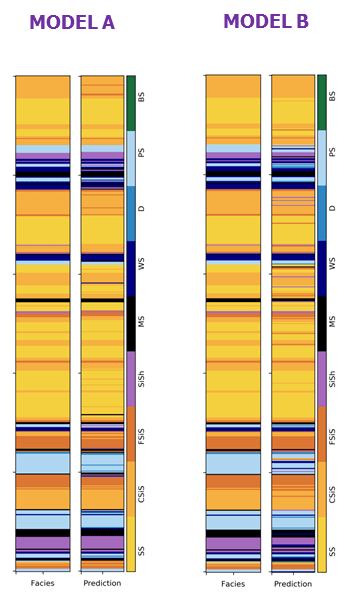Figure 1

As a reminder, both model A and model B were trained to predict the same labeled facies picked by a geologist on core, shown on the left columns (they are identical) of the respective model panels. The right columns in each panels are the predictions.

The question is asked out of context, with no information given about the training process, and or difference in data manipulation (if any) and/or model algorithm used. Very unfair, I know!  And yet, ~78% of 54 respondent clearly indicated their preference for model A. My sense is that this is because model A looks overall smoother and has less of the extra misclassified thin layers.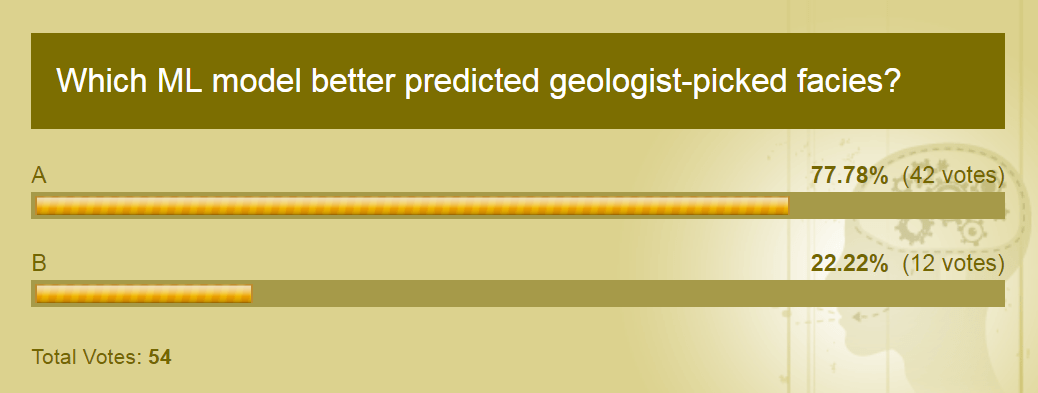Response 1

In part 2, I presented the two predictions, this time accompanied by a the confusion matrix for each model (Figure 2).Figure 2

I asked again which model would be considered better  and this was the result: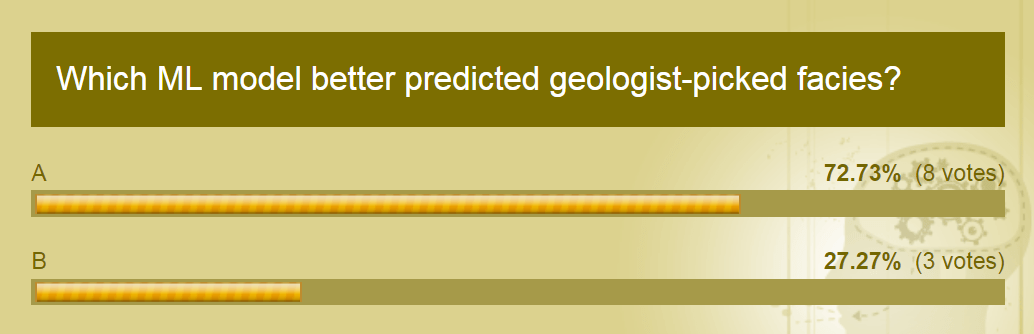Response 2a

Although there were far fewer votes (not as robust a statistical sample) I see that the proportion of votes is very similar to that in the previous response, and decidedly in favor of model A, again. However, the really interesting learning, and to me surprising, came from the next answer (Response 2b): about 82% of the 11 respondents believe the performance scores in the confusion matrix to be realistic.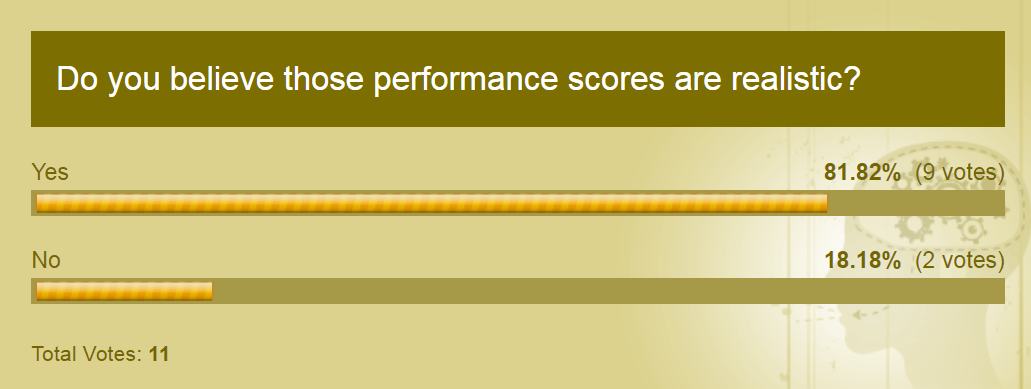Response 2b

Why was it a surprise? It is now time to reveal the trick…..

…which is that the scores in part 2, shown in the confusion matrices of Figure 2, were calculated on the whole well, for training and testing together!!

A few more details:

• I used default parameters for both models
• I used a single 70/30 train/test split (the same random split for both models) with no crossvalidation

which is, in essence, how to NOT do Machine Learning!

In Figure 3, I added a new column on the right of each prediction showing in red which part of the result is merely memorized, and in black which part is interpreted (noise?). Notice that for this particular well (the random 70/30 split was done on all wells together) the percentages are 72.5% and 27.5%.

I’ve also added the proper confusion matrix for each model, which used only the test set. These are more realistic (and poor) results.Figure 3

So, going back to that last response: again, with 11 votes I don’t have solid statistics, but with that caveat in mind one might argue that this is a way you could be ‘sold’ unrealistic (as in over-optimistic) ML results.

At least you could sell them by being vague about the details to those not familiar with the task of machine classification of rock facies and its difficulties (see for example this paper for a great discussion about resolution limitations inherent  in using logs (machine) as opposed to core (human geologist).

#### Acknowledgments

A big thank you goes to Jesper (Way of the Geophysicist) for his encouragement and feedback, and for brainstorming with me on how to deliver this post series.

# Machine Learning quiz – part 2 of 3

In my previous post I posted part 1 (of 3) of a Machine Learning quiz. If you have not read that post, please do, cast your vote, then come back and try part 2 below.

##### QUIZ, part 2

Just as a quick reminder, the image below shows the rock facies predicted from two models, which I just called A and B. Both were trained to predict the same labeled rock facies, picked by a geologist on core, which are shown on the left columns (they are identical) of the respective model panels. The right columns in each panels are the predictions.

*** Please notice that the models in this figure are (very slightly) different from part 1 because I’d forgotten to set the random seed to be the same in the two pipelines (yes, it happens, my apology). But they are not so different, so I left the image in part 1 unchanged and just updated this one.

Please answer the first question: which model predicts the labeled facies “better” (visually)?

Now study the performance as summarized in the confusion matrices for each model (the purple arrows indicate to which model each matrix belongs; I’ve highlighted in green the columns where each model does better, based on F1 (you don’t have to agree with my choice), and answer the second question (notice the differences are often a few 1/100s, or just one).# Variable selection in Python, part I

#### Introduction and recap

In my previous two posts of this (now official, but) informal Data Science series I worked through some strategies for doing visual data exploration in Python, assisted by domain knowledge and inferential tests (rank correlation, confidence, spuriousness), and then extended the discussion to more robust approaches involving distance correlation and variable clustering.

For those that have not read those posts, I am using a dataset comprising 21 wells producing oil from a marine barrier sand reservoir; the data was first published by Lee Hunt in 2013 in a CSEG Recorder paper titled Many correlation coefficients, null hypotheses, and high value.

Oil production, the dependent variable, is measured in tens of barrels of oil per day (it’s a rate, actually). The independent variables are: Gross Pay, in meters; Phi-h, porosity multiplied by thickness, with a 3% porosity cut-off; Position within the reservoir (a ranked variable, with 1.0 representing the uppermost geological facies, 2.0 the middle one, 3.0 the lowest one); Pressure draw-down in MPa. Three additional ‘special’ variables are: Random 1 and Random 2, which are range bound and random, and were included in the paper, and Gross Pay Transform, which I created specifically for this exercise to be highly correlated to Gross pay, by passing Gross pay to a logarithmic function, and then adding a bit of normally distributed random noise.

#### Next step: variable selection (Jupyter Notebooks here)

The idea of variable selection is to try to understand which independent variables are more and which are less important in predicting the dependent variable (Production in this case), and also which ones may be highly correlated to one another (in other words, they carrying the same information); in both cases, assisted by domain knowledge, we drop some of the variables, resulting (ideally) in an improved prediction by a model that is simpler and can generalize better.

I really love the systematic way in which Thomas, working on the same dataset but using R, looked at several methods for variable selection and then summarized all the results in a table. The insight from this (quite) exhaustive analysis helped him chose a subset of variables to use in the final regression. I really, REALLY recommend reading his interactive R notebook.

As for me, one of the goals I had in mind at the end of our 2018 collaboration on this project was to be able to do something similar in Python, and I am delighted to say I think I was able to achieve that goal.

In this post I will look at:

• Distance correlation, again
• Multicollinearity, using Variance Inflation Factor (VIF)
• Sequential feature selection, using both a backward and forward approach
• Random Forest Regressor variable importance, using a drop-column approach
• Multicollinearity, using variable dependence

In the next (1 or 2) post(s) I will look at:

• Permutation importance using an Extra Tree Regressor
• Mutual information
• The relative magnitude of the transformed variables in ACE (Alternating Conditional Expectation)
• SHAP values (Shapley additive explanations)
• The sign of the weights of a neural network (excitory (positive weights) vs. inhibitory (negative weighs))

I think this is a good mix as it combines methods and then summarize the results from all methods.

##### Distance correlation

in Figure 1, below, I plot again the correlation matrix of bivariate scatterplots, rearranged according to the clustering results from last post, and with the distance correlation annotated and coloured by its bootstrapping p-value.

Phi-h, Gross Pay, and Gross pay transform are highly correlation to Production, with statistical significance at the 10%level given by the p-value. However, there is a good chance also also of multicollinearity at play, almost certainly between Gross Pay and Gross Pay Transform, with a DC of 0.97; we know why, in this case, imposed it in this case, but we might have not known.Figure 1. Seaborn pairgrid matrix with distance correlation colored by p-value (gray if > 0.10, blue if p <= 0.10), and plots rearranged by clustering results

##### Variance Inflation Factor (VIF)

Variance inflation factor (VIF) is a technique to estimate the severity of multicollinearity among independent variables within the context of a regression. It is calculated as the ratio of all the variances in a model with multiple terms, divided by the variance of a model with one term alone.

First, we set-up a regression, using the Patsy library to generate design matrices for target and predictors:

```outcome, predictors = dmatrices("Production ~ Gross_pay +Phi_h +Position
+Pressure +Random1 +Random2 +Gross_pay_transform",
data, return_type='dataframe')
```

for which then VIF factors can be calculated with:

```vif["VIF Factor"] = [variance_inflation_factor(predictors.values, i)
for i in range(predictors.shape)]```

The values are summarized in Table I below; variables that have variance inflation factor that is high (ignoring the intercept) and similar in value have a high chance of being collinear because they explain the same variance in the dataset.Table I. Regression VIF factors

For this model, the result suggests either Gross Pay or Gross Pay Transform should be dropped, otherwise the risk is of building a model with high multicollinearity (that is, predictions would be very susceptible to small noise fluctuations).

But which one should we drop? It occurred to me that one possibility would be to drop one in turn and recalculate the VIF factors.Table II. VIF after dropping Gross Pay Transform

As seen in Table II, after removing Gross Pay Transform  all VIF factors are below the cut-off value of 5 (rule-of-thumb suggested in this article, and reference therein). I  would make the additional observation. that because the factors for Phi-h and Gross Pay are now close, even though below the cutoff,  there may be some (smaller amount of) collinearity between the two variables, which is consistent to be expected since both variables contain some information on height (one of pay, one of porosity).

We see something similar when removing Gross Pay; in fact, the Factors for Gross Pay Transform and Phi-h in Table III are also close, yes, but smaller. I’d conclude that VIF is veru sueful in highlighting multicollinearity, but it does not necessarily answer the question of which collinear feature shoud be dropped.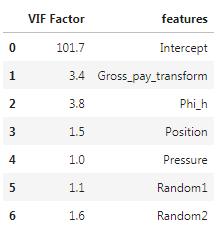Table III. VIF after dropping Gross Pay

##### Sequential feature selection

Sequential feature selection (similarly to Scikit-learn’s Recursive Feature Elimination) is used  “to automatically select a subset of features that is most relevant to the problem. The goal of feature selection is two-fold: we want to improve the computational efficiency and reduce the generalization error of the model by removing irrelevant features or noise”.

I tested both Sequential Forward Selection (SFS) and Sequential Backward Selection (SBS) from Sebastan Raschka‘s  `mlxtend` library to search for that optimal subset of features (for a full overview of the method, and a great set of detailed examples, please see the excellent documentation by Sebastian). You can download and run the full notebook fro the GitHub repo here).

The only difference between SFFS and SBFS is that the former starts with at 1 feature and adds them one by one, whereas the latter starts with all features (or a user defined pre-selected number) and removes them one by one. In both cases I used the selector as part of a pipeline including Scikit-learn’s linear regression and cross-validation with Leave One Out (i.e., dropping one well at a time); for example, the pipeline for SFS is:

```features = data.loc[:, ['Position', 'Gross pay', 'Phi-h', 'Pressure',
'Random 1', 'Random 2', 'Gross pay transform']].values
y = data.loc[:, ['Production']].values

LR = LinearRegression()
loo = LeaveOneOut()

sfs = SFS(estimator=LR,
k_features=7,
forward=True,
floating=True,
scoring='neg_mean_squared_error',
cv = loo,
n_jobs = -1)
sfs = sfs.fit(X, y)
```

and the feature selection results are plotted in Figure 2, generated with a modified version of Sebastian’s `mlxtend` utility function:

```plot_sequential_feature_selection(sfs.get_metric_dict(), kind='std_err')
plt.gca().invert_yaxis()
```

Please notice that having flipped the y axis (my personal preference), performance for SFFS (as given by negative mean square error) improves towards the bottom.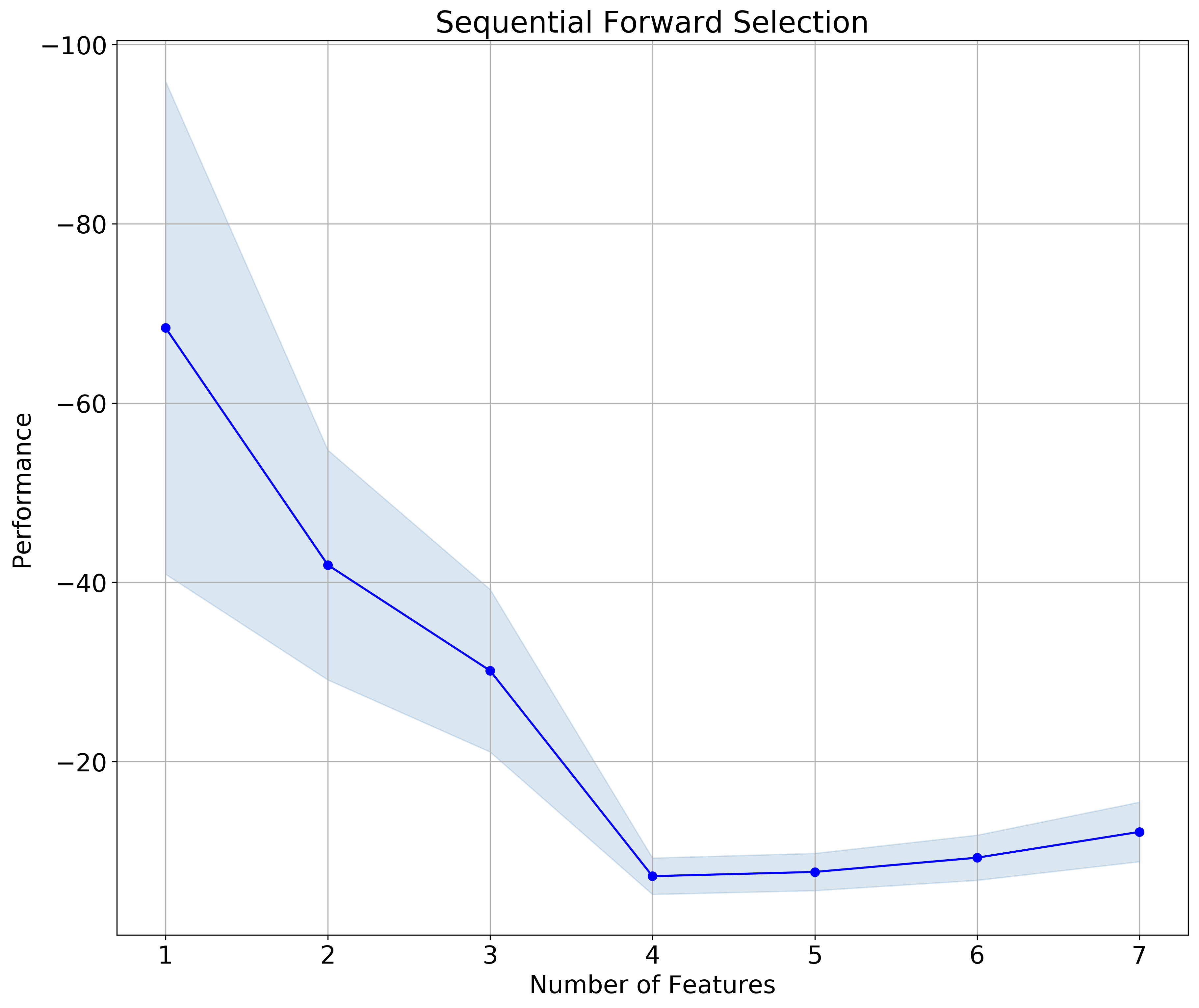Figure 2. Sequential Forward Selection

The results for SFBS is plotted in Figure 3. Notice that in this case I flipped both the y axis  and the x axis; the latter makes the sequential selection go from left to right, which I find a bit more intuitive, given we read from left to right.Figure 3. Sequential Backward Selection

In both cases the subset is made up of 4 feature, and – to my delight !! –  the selected features are the same (check the notebook to see how I extract the information):

```>>>  ['Position', 'Gross pay', 'Phi-h', 'Pressure']
```
##### Drop-column feature importance

You can download the notebook for both drop-column importance and dependence from here.

I have to say I’ve never been comfortable with using Feature Importance plots you get from Random Forest. In part because, on occasion, I noticed a disconnect with what domain knowledge-informed intuition would suggest; in part, I confess, because I thought (and I was right) I had an incomplete understanding of what goes on in the background. Until I read the article How to not use random forest. The example with toy dataset in there is not the most exciting, but it demonstrate clearly how using Feature Importance with preset parameters places a random variable at the top. If you wonder how can that be, I recommend reading the article.

Or read on, there’s more coming: curious, I did some more searching, and found this article, Selecting good features – Part III: random forests. There’s a nicer example in there, using the Boston Housing dataset, and to me a clearer explanation of why one should not use the default Scikit-learn Mean Decrease Impurity metric (strong, but correlated features can end up with low scores).

Finally, I found Beware Default Random Forest Importances, where the authors (thank you!!!) not only walk readers through a full set of experiments, run in both Python and R, but provide a great library (called `rfpimp`), to do your own work in Python.

I really like their drop-column importance, which is implemented to answers the question of how important a feature is to the overall model performance … and does it  … even more directly than the permutation importance.

That is achieved with a brute force drop-column apprach involving:

• training the model with all features to get a baseline performance score
• dropping a column
• retraining  the model and recomputing the performance score.

The importance value of a feature is then the difference between the baseline and the score from the model without that feature.

I also REALLY like that unimportant features do not have just very low importance; some do, but some have negative importance, exposing that removing them improves model performance. This is the case, with our small dataset of the Random 1 and Random 2 variables, as shown in Figure 4. It is also the case of Pressure. Of the remaining variables, Gross Pay Transform has very low importance (please notice the range is 0-0.15 for this plot, a conscious choice by the authors), Gross pay and Phi-h look somewhat important, and Position in the reservoir is the most important feature. This is excellent insight; please compare to the importances with Scikit-learn’s defautl metric, in Figure 5.Figure 4. rfpimp Drop-column importance. Notice the 0-0.15 range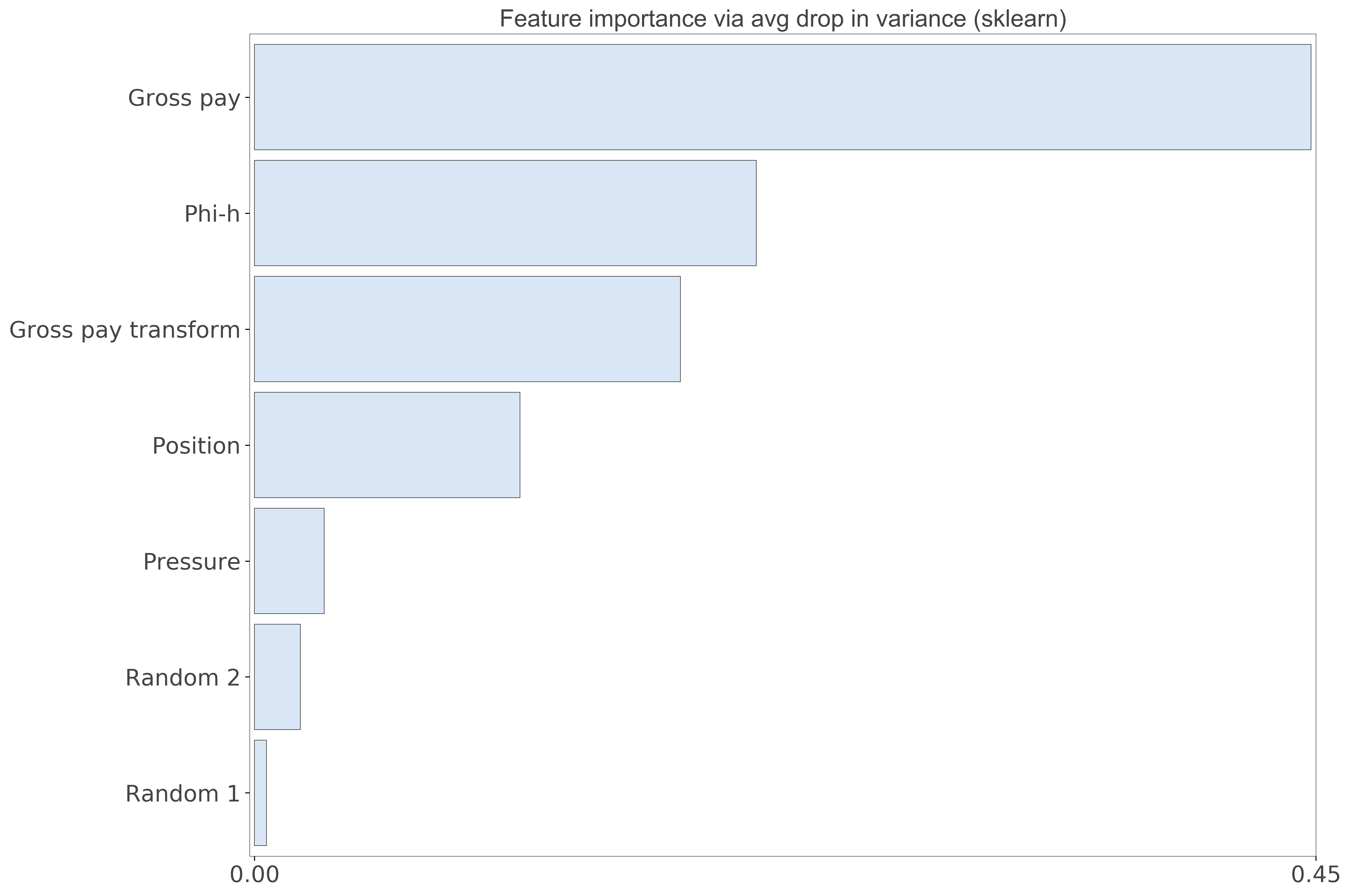Figure 5. Scikit-learn Feature importance. Notice the 0-0.45 range

##### Dependence

This last analysis is similar to Thomas’ Redundancy Analysis in that we look for those variables that can be predicted using the other variables.  Using the `feature_dependence_matrix` function from the `rfpimp` library we get:

```>>> Dependence:
Gross pay               0.939
Gross pay transform     0.815
Phi-h                   0.503
Random 2               0.0789
Position               0.0745
Pressure               -0.396
Random 1               -0.836

```

By removing Gross Pay Transform, and repeating the analysis, we get:

```>>> Dependence:
Gross pay    0.594
Phi-h        0.573
Random 2     0.179
Position     0.106
Pressure    -0.339
Random 1    -0.767```

and by removing Gross Pay:

```>>> Dependence:
Gross pay transform     0.479
Phi-h                   0.429
Position                0.146
Random 2              -0.0522
Pressure               -0.319
Random 1               -0.457

```

These results show, again, that either Gross Pay or Gross Pay Transform should be dropped (perhaps the former), because of very high chance of dependence (~multicollinearity). Also Phi-h is somewhat predictable from the other variables, but not as much, so it may be fine, if not good, to keep it (that’s what domain knowledge would suggest).

They are in agreement with the results from VIF, but this time the outcome is blind to the outcome (the target Production) so I’d consider it more robust.

# Machine Learning quiz – part 1 of 3

#### Introduction

I’ve been meaning to write about the 2016 SEG Machine Learning Contest for some time. I am thinking of a short and not very structured series (i.e. I’ll jump all over the place) of 2, possibly 3 posts (with the exclusion of this quiz). It will mostly be a revisiting – and extension – of some work that team MandMs (Mark Dahl and I) did, but not necessarily posted. I will touch most certainly on cross-validation, learning curves, data imputation, maybe a few other topics.

#### Background on the 2016 ML contest

The goal of the SEG contest was for teams to train a machine learning algorithm to predict rock facies from well log data. Below is the (slightly modified) description of the data form the original notebook by Brendon Hall:

The data is originally from a class exercise from The University of Kansas on Neural Networks and Fuzzy Systems. This exercise is based on a consortium project to use machine learning techniques to create a reservoir model of the largest gas fields in North America, the Hugoton and Panoma Fields. For more info on the origin of the data, see Bohling and Dubois (2003) and Dubois et al. (2007).

This dataset is from nine wells (with 4149 examples), consisting of a set of seven predictor variables and a rock facies (class) for each example vector and validation (test) data (830 examples from two wells) having the same seven predictor variables in the feature vector. Facies are based on examination of cores from nine wells taken vertically at half-foot intervals. Predictor variables include five from wireline log measurements and two geologic constraining variables that are derived from geologic knowledge. These are essentially continuous variables sampled at a half-foot sample rate.

The seven predictor variables are:

The nine discrete facies (classes of rocks) are:For some examples of the work during the contest, you can take a look at the original notebook, one of the submissions by my team, where we used Support Vector Classification to predict the facies, or a submission by the one of the top 4 teams, all of whom achieved the highest scores on the validation data with different combinations of Boosted Trees trained on augmented features alongside the original features.

##### QUIZ

Just before last Christmas, I run a little fun experiment to resume work with this dataset. I decided to turn the outcome into a quiz.

Below I present the predicted rock facies from two distinct models, which I call A and B. Both were trained to predict the same labeled facies picked by the geologist, which are shown on the left columns (they are identical) of the respective model panels. The right columns in each panels are the predictions. Which predictions are “better”?

Please be warned, the question is a trick one. As you can see, I am gently leading you to make a visual, qualitative assessment of “better-ness”, while being absolutely vague about the models and not giving any information about the training process, which is intentional, and – yes! – not very fair. But that’s the whole point of this quiz, which is really a teaser to the series.